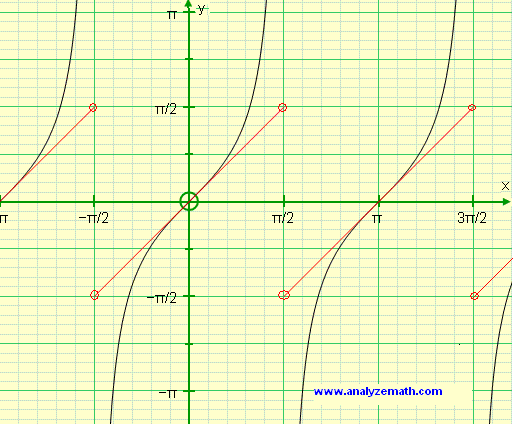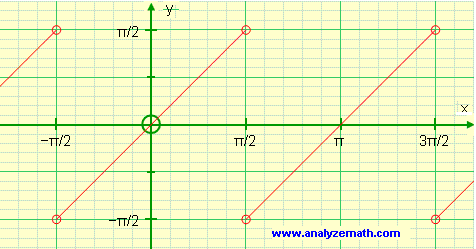# Find Derivative of f(x) = arctan(tan(x)) and graph it

A calculus tutorial on how to find the first derivative of f(x) = arctan(tan(x)) and graph f and f ' in its natural domain.

## Graphs of tan(x) and arctan(tan(x))

f(x) is defined for all values x in R except x = π/2 + k * π, where k is an integer. Since tan(x) is periodic, then f(x) = arctan(tan(x)) is also a periodic function.
As x increases from -π/2 to π/2 exclusive, tan(x) increases from infinitely small values (- infinity) to infinitely large values (+ infinity) and arctan(tan(x)) increases from -π/2 to π/2 exclusive since tan(x) is undefined at -π/2 and + π/2. In fact for x in (-π/2 , π/2) arctan(tan(x)) = x.
Since tan(x) has a period of &π;, arctan(tan(x)) also has a period of π. The graph below shows the graphs of arctan(tan(x)) and tan(x) from -π/2 to 3π/2.Domain of f: R - {π + k*π/2 , k integer}
Range of f: (-π/2 , π/2)
The graph below shows the graphs of arctan(tan(x)).## Derivative of f(x) = arctan(tan(x)) and its Derivative

f(x) is a composite function and the derivative is computed using the chain ruleas follows: Let u = tan(x)
Hence f(x) = arctan(u(x))
Apply the chain rule of differentiation
f '(x) = du/dx d(arctan(u))/du = (1 / cos(x)
2) * 1 / (u2) + 1)
= (1 / cos(x)
2) * 1 / (tan(x)2(x) + 1)
= 1 , for x not equal to π/2 + k*π/2 where k is an integer.
Below is shown arctan(tan(x)) in red and its derivative in blue. Note that the derivative is undefined for values of x for which cos(x) is equal to 0, which means at x = π/2 + k * π, where k is an integer. Note that f(x) is undefined for these same values of x.